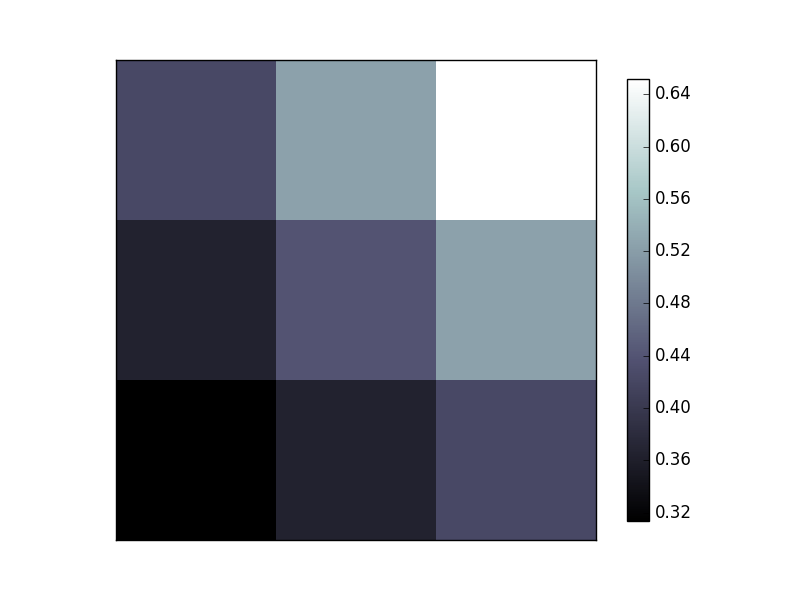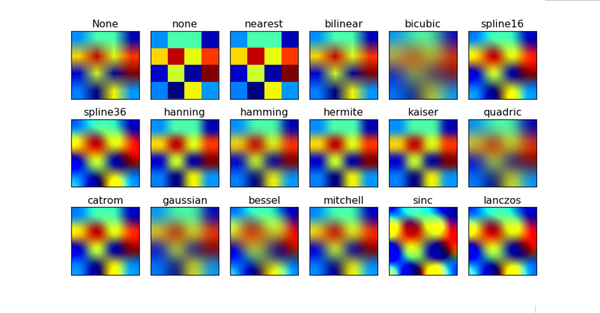# Matplotlib Image 图片

674℃

## 随机矩阵画图

``````import matplotlib.pyplot as plt
import numpy as np

a = np.array([0.313660827978, 0.365348418405, 0.423733120134,
0.365348418405, 0.439599930621, 0.525083754405,
0.423733120134, 0.525083754405, 0.651536351379]).reshape(3,3)````````plt.imshow(a, interpolation='nearest', cmap='bone', origin='lower')``

## 出图方式## colorbar

``````plt.colorbar(shrink=.92)

plt.xticks(())
plt.yticks(())
plt.show()``````